International Journal of Astrophysics and Space Science
Volume 3, Issue 4, August 2015, Pages: 60-64

Evaluation of the Average Energy Density of the Electromagnetic Component of the Physical Vacuum and Quantum Nature of Gravity

Timashev Serge1, 2, 3

1Institute of Functional Nuclear Electronics, National Research Nuclear University MEPhI, Moscow, Russia

2Laboratory of Theory of Physicochemical Processes, Karpov Institute of Physical Chemistry, Moscow, Russia

3Laboratory of Laser Chemistry, Institute of Laser and Information Technology, RAS, Moscow, Russia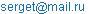Timashev Serge. Evaluation of the Average Energy Density of the Electromagnetic Component of the Physical Vacuum and Quantum Nature of Gravity. International Journal of Astrophysics and Space Science. Vol. 3, No. 4, 2015, pp. 60-64. doi: 10.11648/j.ijass.20150304.12

Abstract: In the frame of the previously proposed model of the Universe dynamics (Timashev Serge. Planckian Energy-Mass Source and the Dynamics of the Universe: Phenomenology // International Journal of Astrophysics and Space Science, 2014, Vol. 2, No. 3, pp. 33-45), the substance of dark energy is associated with the electromagnetic component of the physical vacuum (EM vacuum). The estimate of this value obtained in this paper shows that the calculated zero-point oscillation energy does indeed constitute dark energy with the density established by the present time. At the same time, the energy density of the ЕМ-vacuum completely defines the dimensionless constant of gravitational interactions, demonstrating the quantum nature of gravity.

Keywords: Dark Energy, Electromagnetic Component of the Physical Vacuum, Cosmological Constant, Elementary Gravitational Mass, Planck Angular Frequency, Quantum Gravity

Contents

1. Introduction

It is assumed, in accordance with the standard model of the Universe dynamics [1-3] that dark energy density, which determines the cosmological constant L according to the relation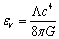(1)

where G is the gravitational constant and c is the velocity of light in vacuum, is spread uniformly all over the Universe. It is believed that dark energy is evenly "spilled" over the Universe and satisfies the equation of state pV/eV = –1, with the pressure pV being negative. It is precisely this relationship, sort of implying antigravity of dark energy, that manifests itself as the factor governing the expansion of the Universe, the questions as to the physically perceptible nature of such repulsive interactions remaining open at that. The problems arising within the scope of the standard model, in addition to those associated with the introduction of the debatable entities – dark energy and dark matter, are accentuated because of the unsuccessful attempts [1-3] to correlate the quantity eV ≈ 0.66×10–8 erg/cm3 observed  with the parameters of the physical vacuum. The difference comes to more than many orders of magnitude. Such catastrophic differences, such an "orders-of-magnitude discrepancy", are considered to be a "severe trial for the entire fundamental theory" .

Within the context of the ideas put forward in [5, 6], the substance of dark energy is identified with the electromagnetic component of the physical vacuum (EM vacuum) which is spread uniformly all over the base space of the Universe expanding in compliance with the Friedmann equation and characterized by the global time common to all points of space that is reckoned from the instant the Big Bang had taken place [2, 7]. It should be noted here that according to  the Universe is an open system, and the constantly acting hypothetical source of Planckian power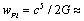1.8´1059 erg/s,           (2)

which originated at the onset of the inflatory phase of the Big Bang , could produce the entire energy-mass of the observable Universe during the course of its evolution. We will assume that the energy of this hypothetical source of Planckian power is being uniformly distributed and generated constantly in every element of the already originated and originating space, every element of the Universe’s volume expanding as a result of such an energy release, though actual manifestations of this expansion can only be detected on cosmological scales.

To go over to more adequate models of the dynamics of the Universe that would allow for nonuniformity in the distribution of masses over space, would require introduction of notions about successive origination of local Planckian power sources that integrally maintain the conservation of the fundamental cosmological principle – the homogeneity and isotropism of the distribution of matter over the Universe . Considered as one such possibility may be a temporally consecutive switching-on of Planckian energy-mass sources of chaotically varying localization on the outer sphere of the Hubble radius RH; i.e., at the boundary between the already formed Universe and the primeval, inflation vacuum whose space had begun being absorbed, following the Big Bang, by the expanding Universe being formed. In other words, we will assume that in contrast to the existing models of the dynamics of the Universe [9, 10] not all of the energy of the inflation vacuum, defined as the "false", metastable vacuum with an energy density exceeding that of the vacuum of the Universe, had been released within a very short time, a tiny fraction of a second following the Big Bang and realized as the observable energy of the Universe. We will take it that the process of such an "assimilation" of the energy of the false vacuum and its concurrent transformation into the energy of the expanding Universe runs incessantly during the course of the fluctuation-induced origination of Planckian power sources in the neighborhood of the boundary between the two vacua, namely, the physical and the false vacuum.

Therefore, the total energy of the Universe is being produced and constantly "fed to" by the energy of the inflation vacuum. Such "feeding" is necessitated by the dissipation resulting from various processes initiated by the EM vacuum (the formation of new volumes of the Universe during its expansion, in addition to the dissipative processes – spontaneous emission, Lamb shift, contribution to the radiation damping force, dynamical Casimir effect, etc., taking place in the entire Universe) [5, 6, 11-13]. The phenomenological concepts being developed in [5, 6] have made it possible to resolve the problems arising in the standard theory [1-3], those associated with the introduction of "dark energy" and "dark matter" included, from a unified position – with the Planckian energy-mass source introduced into the dynamics of the Universe and the base reference system with common global time used. The EM vacuum here also plays the part of the substance that unites and forms all the known types of interaction – strong, electromagnetic, weak, and gravitational . Below will also be shown that calculated zero-point oscillation energy of EM vacuum does actually constitute dark energy with the density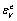established by the present time  (the superscript points to the connection between dark energy and the EM vacuum).

2. Zero-Point Energies of the EM Field Oscillators

It has been well known  that any solution of the electromagnetic field (EMF) equations can be represented, thanks to their linearity, in the form of superposition of monochromatic waves that can be treated as individual EMF degrees of freedom characterized by their cyclic frequencies ω. The total energy of such a distributed system can be represented as a sum of energies of the field oscillators; and for the average energy accounted for by the frequency interval from ω to ω + dω the following relation holds true :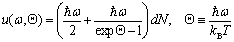(3)

where kB and T are the Boltzmann constant and absolute temperature, respectively,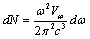is the number of field oscillators whose frequencies fall within the above-mentioned interval, and Vω is the configurational volume occupied by the field corresponding to the oscillator with the frequency ω. The right-hand side of expression (3) contains two qualitatively different terms. If the "dark energy" associated with the first term is uniformly spread over the base space of the Universe, the EMF associated with the second term (the Planck blackbody spectral distribution) bears a certain local relationship to the environmental conditions – a concrete temperature.

Our objective consists in the finding of the total energy density, which is attained by integrating expression (3) over the entire interval of possible frequencies and relating the energy thus obtained to unit volume. In doing so, one should bear in mind that regions of constant temperature are chaotically scattered about the Universe and have different extents. Therefore, when calculating the radiant energy density component associated with the second term, it proves convenient to relate it solely to one such region and choose the configurational volume as some constant quantity, Vw = V = const, assuming that the filed formed by the oscillators with all the possible frequencies is localized within this volume. The main contribution in the integration of the second term is obviously due to the frequency region in the neighborhood of the maximum of the Planck function, at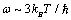, and so integration may formally be over an infinite frequency interval. As a result, considering the fact that each wave vector is associated with two polarization states, we get the following expression for the Planck radiant energy density eP :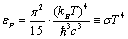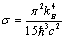(4)

When determining the density of the EM vacuum energy component – the zero-point oscillation energy, which is obtained by integrating the first term on the right-hand side of expression (3) and relating the energy obtained to unit volume, one should bear in mind the following. First of all, on the basis that dark energy is evenly distributed over the entire space in the Universe, whose extent is characterized by the Hubble radius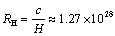cm, where H is the Hubble constant, the total zero-point oscillation energy should be averaged over a volume of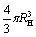(see expression (5) in ). The main contribution to the total zero-point EMF oscillation energy is due to the highest frequencies, beginning with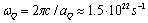, where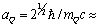1.3 10 –13 cm is the "Bohr radius" as a spatial scale associated with the "elementary gravitational mass" mQ . Such frequencies as correspond to the intranuclear excitations, EQCD = mQc2 ≈ 209.5 MeV, are critical for phase transition: quarks within the nucleus are no longer bound in nucleons, so that there forms quark-gluon plasma. It is but natural to consider the Planck angular frequency ωPl as the maximum possible cyclic frequency of the zero-point EMF oscillations :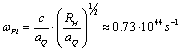.

The spatial scale corresponding to this field is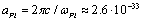cm.

Since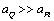, we take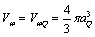as the configurational volume in calculating the average zero-point EMF oscillation energy density. In that case, when integrating the first term on the right-hand side of expression (3) over the frequency interval [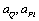] and relating the energy obtained to unit volume (the factor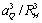), we get, with due regard for the fact that each wave vector is associated with two polarization states,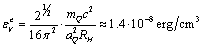(5)

The quantity found (considering certain conditionality in selecting the numerical coefficients when introducing the parameters used) is practically close to the above-indicated quantity obtained on the basis of observation data.

3. Quantum Nature of Gravity

For subsequent assessments it is convenient to introduce the dimensionless ratio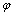of the energy densityof the ЕМ vacuum to the density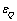of intranuclear excitations EQCD = mQc2, localized in the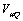volume: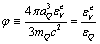(6)

In accordance with Eqs. (5)-(6) and Eq. (12) of paper , the dimensionless gravitational interaction constant αg can be presented as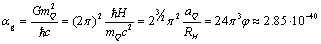(7)

The dependence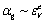means the quantum nature of gravity only. This is in complete accordance with the concepts [5, 6], according to which the EM vacuum is the basic medium for the material objects and is polarized in the vicinity of these facilities. Indeed, according to , the potential energy of the EM vacuum polarization in the vicinity of a particle of mass m0 looks as an attractive potential energy: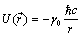(8)

Here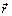denotes the radius-vector (the origin of coordinates coincides with the particle’s position);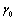is a dimensionless parameter, which reflect the degree of connection between a particle of mass m0 and the electromagnetic component of the physical vacuum subject to polarization. The position of the lower energetic level, which characterizes the binding energy between the particle under consideration and the EM-vacuum (we believe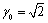), corresponds in magnitude to the "rest energy" of the particle in the form suggested by Einstein: E0 = mc2. In that case,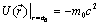. It follows from the meaning of this expression that it would be more proper to refer to the quantity E0 as the "binding energy between the particle and the EM vacuum" than the "rest energy of the particle", the mass defect in nuclear processes simply characterizing the energy released as a result of the difference in energy between the bindings of the original and final products with the vacuum. The localization region (the "Bohr radius") of the particle in this case,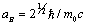, is considered as the characteristic spatial scale of the introduced "vacuum polaron". Note also, the polarization of the medium can affect the dynamics of a particle with a mass of m0 moving freely in it at a velocity of u. It is precisely because the rate of alteration of the physical vacuum surrounding a moving object is limited to the value of c that the movement of material bodies with velocities approaching c in this vacuum proves impossible .

Naturally, at distances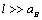from the particles on which the gravitational effects usually are analyzed, the initial expression (8) for the potential energy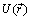of the EM vacuum in the vicinity of the particle of mass m0 must be supplemented by introducing the "gravitational" permittivity ηg as a phenomenological factor to the denominator. It is natural to assume that it is a factor leading to the formation of a well-known gravitational potential energy: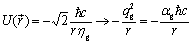(9)

Here qg2GmQ2 is the squared elementary "gravitational" charge . Then in view of (6) we obtain: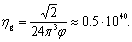(10)

It is natural to assume the "gravitational" permittivity ηg is a factor leading to the formation of a well-known gravitational potential energy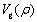between two masses m1 and m2, located at a distance ρ from each other: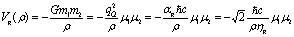(11)

Here μi = mi/mQ is the relative mass of the ith particle. Here, it is assumed that the degeneracy resulting from potential energy indistinguishability (8) in the vicinity of each mass is lifted due to the gravitational interaction with permittivity ηg, and the potential energy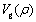now includes multipliers μ1μ2.

It is believed that the abnormally high value of the gravitational permittivity is a physical cause of the smallness of gravitational interactions, which is formally, in accordance with Eq. (7), associated with the ratio between the characteristic size of the polarization region of the EM vacuum in the vicinity of the particle of "elementary gravitational mass" and the characteristic size of the Universe. It will be estimated that the gravitational field is not substantially attenuated by the EM vacuum environment.

4. Concluding Remarks

EM vacuum is not only the basic environment for all material objects, but also the environment, actively forming and uniting all four fundamental interactions – gravitational, electromagnetic, strong nuclear and weak nuclear interaction. Quantum nature of gravitational interaction is set higher. In essence, the gravitational interaction does not have its specifics. It is a consequence of the polarization of the EM vacuum in the vicinity of any mass for an appropriate pairing of EM vacuum components and their derivatives on both sides of the interface [15, 16], as well as extremely high gravitational permittivity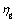. Such high value can be attributed to the integrity of the EM vacuum as a system in which all the coupled areas of any space scales are dynamically related through the fluctuating values of the squares of the electric and magnetic fields on all possible frequency bands.

According to [5, 16], the physical unity of the electromagnetic and strong interactions at the phenomenological level is detected in the analysis of the polarization of the EM vacuum by hadrons with the conjugation of the normal component of the electric field vector and the normal derivatives on the boundary conditions of hadrons with EM vacuum.

The intrinsic affinity between the electromagnetic and weak interactions, whose vehicles are vector bosons – photons and heavy bosons, respectively, is of different nature. These interactions prove to be a unified electroweak interaction only at energies on the order of 100 GeV, which is commensurable with the rest energy of intermediate vector bosons . However, one can assume that it is the fluctuations of EM vacuum field, associated with the intrinsic dynamics of nuclei, that initiate the emergence of virtual vector bosons and effective realization of four-fermion interactions leading to weak nuclear processes at low energies. We present here the quantity squared "elementary weak interaction charge"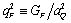, whereis the Fermi four-fermion interaction constant , and the corresponding dimensionless constant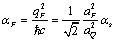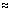(12)

where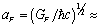0.69·10 – 16 cm.

Thus, here is in fact introduced some generalized image of the electromagnetic component of the physical vacuum that involves a substantial proportion of the entire energy of the Universe and initiates manifestation of strong and weak interactions by exerting effect on every nucleus as an open system.

Finally, we also note that from relations (1) and (5) there follows the representation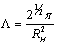(12)

that can be handy for the understanding of the physical essence of the cosmological constant. Note that in accordance with the general ideas of  it follows from expression (9) that the cosmological constant decreases as the Universe expands, which disagrees with the generally accepted conclusion that the expansion of the Universe is accelerating.

Acknowledgements

The author thanks J. Botha and B.M. Grafov for useful discussions.

References

1. Sahni V. Dark matter and dark energy // Lect. Notes Phys. 2004.V. 653. P. 141-180; arxiv.org/abs/astro-ph/0403324v3.
2. Lukash V.N., Rubakov V.A.Dark energy: myths and reality // Physics – Uspekhi. Advances in Physical Sciences. 2008. V. 51. N 3. P. 283–289.
3. Chernin A.D. Dark energy and universal antigravitation // Physics – Uspekhi. Advances in Physical Sciences. 2008. V. 51. N 3. P. 253–282.
4. Padmanabhan T. Darker side of the Universe. 29 International Cosmic Ray Conference Pune. 2005. V. 10. P. 47-62.
5. Timashev Serge. Dynamical essence of the basic relations of the special theory of relativity and the origin of fundamental interactions: Phenomenology // International Journal of Astrophysics and Space Science, 2014. V. 2. N. 2. P. 22-32
6. Timashev Serge. Planckian energy-mass source and the dynamics of the Universe: Phenomenology // International Journal of Astrophysics and Space Science, 2014. V. 2. N. 3. P. 33-45.
7. Burlankov D.E. Time, space, gravity. Moscow-Izhevsk: NIC "Regular and Chaotic Dynamics". 2006. 420 p. (in Russian)
8. Klapdor-Kleingrothaus H.V., Zuber K. Teilchenastrophysik. B.G. Teubner GmbH, Stuttgart, 1997.
9. Vilenkin A. Many worlds in one: The search for other universes. New York: Hill and Wang. 2006, 235 p.
10. Linde A. Inflation, Quantum Cosmology and the Anthropic Principle. In: "Science and Ultimate Reality: From Quantum to Cosmos", honoring John Wheeler’s 90th birthday. J. D. Barrow, P.C.W. Davies, & C.L. Harper eds. Cambridge: Cambridge University Press, 2003
11. Efremov G.F., Sharkov V.V., Krupennikov D.V. Nondivergent statistical quantum electrodynamics // International Journal of Bifurcation and Chaos, 2008. V. 18. N. 9. P. 2817-
12. Dalvit D.A.R., Neto P.A.M., Mazzitelli F.D. Fluctuations, dissipation and the dynamical Casimir effect. http://arxiv.org/abs/1006.4790v2.
13. Wilson C.M., Johansson G., Pourkabirian A., Johansson J.R., Duty T., Nori F., Delsing P. Observation of the Dynamical Casimir Effect in a Superconducting Circuit. http://arxiv.org/pdf/1105.4714v1
14. Terletsky Ya.P. Statistical Physics (in Russian). Moscow: Vysshaya Shkola. 1994. 353 p.
15. Milton K.A., DeRaad L.L., Jr, Schwinger J. Casimir self-stress on a perfectly conducting spherical shell // Annals of Physics. 1978. V. 115. P. 388-403.
16. Timashev S.F. Radioactive decay of nuclei as a nuclear-chemical process initiated by electrons // Russian J. Phys. Chemistry A. 2015. V. 89. N. 11.
17. Bettini A. Introduction to elementary particle Physics. Cambridge: Cambridge University Press. 2008, 431 p.

 Contents 1. 2. 3. 4.
Article ToolsAbstractPDF(191K)